GATE  >  Biotechnology - (BT) 2018 GATE Paper (Practice Test)

# Biotechnology - (BT) 2018 GATE Paper (Practice Test)

Test Description

## 65 Questions MCQ Test GATE Past Year Papers for Practice (All Branches) | Biotechnology - (BT) 2018 GATE Paper (Practice Test)

Biotechnology - (BT) 2018 GATE Paper (Practice Test) for GATE 2023 is part of GATE Past Year Papers for Practice (All Branches) preparation. The Biotechnology - (BT) 2018 GATE Paper (Practice Test) questions and answers have been prepared according to the GATE exam syllabus.The Biotechnology - (BT) 2018 GATE Paper (Practice Test) MCQs are made for GATE 2023 Exam. Find important definitions, questions, notes, meanings, examples, exercises, MCQs and online tests for Biotechnology - (BT) 2018 GATE Paper (Practice Test) below.
Solutions of Biotechnology - (BT) 2018 GATE Paper (Practice Test) questions in English are available as part of our GATE Past Year Papers for Practice (All Branches) for GATE & Biotechnology - (BT) 2018 GATE Paper (Practice Test) solutions in Hindi for GATE Past Year Papers for Practice (All Branches) course. Download more important topics, notes, lectures and mock test series for GATE Exam by signing up for free. Attempt Biotechnology - (BT) 2018 GATE Paper (Practice Test) | 65 questions in 180 minutes | Mock test for GATE preparation | Free important questions MCQ to study GATE Past Year Papers for Practice (All Branches) for GATE Exam | Download free PDF with solutions
 1 Crore+ students have signed up on EduRev. Have you?
Biotechnology - (BT) 2018 GATE Paper (Practice Test) - Question 1

### “When she fell down the _______, she received many _______ but little help.” The words that best fill the blanks in the above sentence are

Biotechnology - (BT) 2018 GATE Paper (Practice Test) - Question 2

### “In spite of being warned repeatedly, he failed to correct his _________ behaviour.” The word that best fills the blank in the above sentence is

Biotechnology - (BT) 2018 GATE Paper (Practice Test) - Question 3

### For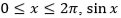and cos x are both decreasing functions in the interval ________.

Biotechnology - (BT) 2018 GATE Paper (Practice Test) - Question 4

The area of an equilateral triangle is √3. What is the perimeter of the triangle?

Biotechnology - (BT) 2018 GATE Paper (Practice Test) - Question 5

Arrange the following three-dimensional objects in the descending order of their volumes:

(i) A cuboid with dimensions 10 cm, 8 cm and 6 cm

(ii) A cube of side 8 cm

(iii) A cylinder with base radius 7 cm and height 7 cm

(iv) A sphere of radius 7 cm

Biotechnology - (BT) 2018 GATE Paper (Practice Test) - Question 6

An automobile travels from city A to city B and returns to city A by the same route. The speed of the vehicle during the onward and return journeys were constant at 60 km/h and 90 km/h, respectively. What is the average speed in km/h for the entire journey?

Biotechnology - (BT) 2018 GATE Paper (Practice Test) - Question 7

A set of 4 parallel lines intersect with another set of 5 parallel lines. How many parallelograms are formed?

Biotechnology - (BT) 2018 GATE Paper (Practice Test) - Question 8

To pass a test, a candidate needs to answer at least 2 out of 3 questions correctly. A total of 6,30,000 candidates appeared for the test. Question A was correctly answered by 3,30,000 candidates. Question B was answered correctly by 2,50,000 candidates. Question C was answered correctly by 2,60,000 candidates. Both questions A and B were answered correctly by 1,00,000 candidates. Both questions B and C were answered correctly by 90,000 candidates. Both questions A and C were answered correctly by 80,000 candidates. If the number of students answering all questions correctly is the same as the number answering none, how many candidates failed to clear the test?

Biotechnology - (BT) 2018 GATE Paper (Practice Test) - Question 9

If ��2 + �� − 1 = 0 what is the value of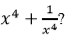?

Biotechnology - (BT) 2018 GATE Paper (Practice Test) - Question 10

In a detailed study of annual crow births in India, it was found that there was relatively no growth during the period 2002 to 2004 and a sudden spike from 2004 to 2005. In another unrelated study, it was found that the revenue from cracker sales in India which remained fairly flat from 2002 to 2004, saw a sudden spike in 2005 before declining again in 2006. The solid line in the graph below refers to annual sale of crackers and the dashed line refers to the annual crow births in India. Choose the most appropriate inference from the above data.

.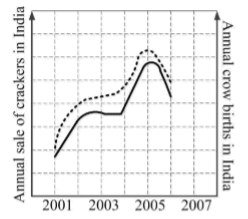Biotechnology - (BT) 2018 GATE Paper (Practice Test) - Question 11

Consider an unfair coin. The probability of getting heads is 0.6. If you toss this coin twice, what is the probability that the first or the second toss is heads?

Biotechnology - (BT) 2018 GATE Paper (Practice Test) - Question 12

If serum is removed from the growth medium of human embryonic kidney cell line (HEK), then the cells will

Biotechnology - (BT) 2018 GATE Paper (Practice Test) - Question 13

The repeat sequence of telomere in humans is

Biotechnology - (BT) 2018 GATE Paper (Practice Test) - Question 14

If a segment of a sense strand of DNA is 5'-ATGGACCAGA-3', then the resulting RNA sequence after transcription is

Biotechnology - (BT) 2018 GATE Paper (Practice Test) - Question 15

Which one of the following is an example of a neurotoxin?

Biotechnology - (BT) 2018 GATE Paper (Practice Test) - Question 16

Which of the following components constitute a molecular mechanics force field?

P. Bond stretching
Q. Bond angle bending
R. Torsional bond rotation
S. Non-bonded interactions

Biotechnology - (BT) 2018 GATE Paper (Practice Test) - Question 17

Which one of the following BLAST search programs is used to identify homologs of a genomic DNA query in a protein sequence database?

Biotechnology - (BT) 2018 GATE Paper (Practice Test) - Question 18

A mixture contains three similarly sized peptides P, Q and R. The peptide P is positively charged, Q is weakly negative and R is strongly negative. If this mixture is passed through an ion-exchange chromatography column containing an anionic resin, their order of elution will be

Biotechnology - (BT) 2018 GATE Paper (Practice Test) - Question 19

Which one of the following is INCORRECT about protein structures?

Biotechnology - (BT) 2018 GATE Paper (Practice Test) - Question 20

Which one of the following metabolic processes in mammalian cells does NOT occur in the mitochondria?

Biotechnology - (BT) 2018 GATE Paper (Practice Test) - Question 21

Which one of the following is NOT a principal component of innate immunity?

Biotechnology - (BT) 2018 GATE Paper (Practice Test) - Question 22

Which of the following technique(s) can be used to study conformational changes in myoglobin?
P. Mass spectrometry
Q. Fluorescence spectroscopy
R. Circular dichroism spectroscopy
S. Light microscopy

Biotechnology - (BT) 2018 GATE Paper (Practice Test) - Question 23

Which one of the following bioreactor configurations is the basis for a trickling biological filter?

Biotechnology - (BT) 2018 GATE Paper (Practice Test) - Question 24

Cell type A secretes molecule X into the culture medium. Cell type B in the same culture responds to the molecule X by expressing protein Y. Which one of the following modes of signaling represents the interaction between A and B?

Biotechnology - (BT) 2018 GATE Paper (Practice Test) - Question 25

Which one of the following statements is true for actin?

Biotechnology - (BT) 2018 GATE Paper (Practice Test) - Question 26

Standard error is

Biotechnology - (BT) 2018 GATE Paper (Practice Test) - Question 27

Which one of the following techniques is used to monitor RNA transcripts, both temporally and spatially?

Biotechnology - (BT) 2018 GATE Paper (Practice Test) - Question 28

Identify the character based method(s) used for the construction of a phylogenetic tree.
P. Maximum parsimony
Q. Neighbor joining
R. Maximum likelihood
S. Bootstrapping

Biotechnology - (BT) 2018 GATE Paper (Practice Test) - Question 29

Which one of the following is the solution for cos2�� + 2cosx + 1 = 0, for values of �� in the range of 0° < �� < 360°?

Biotechnology - (BT) 2018 GATE Paper (Practice Test) - Question 30

Which one of the following plant secondary metabolites is a natural insecticide?

Biotechnology - (BT) 2018 GATE Paper (Practice Test) - Question 31

The determinant of the matrix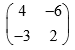_____________________.

Biotechnology - (BT) 2018 GATE Paper (Practice Test) - Question 32

Protein binding regions of DNA are identified by one of the following techniques

Detailed Solution for Biotechnology - (BT) 2018 GATE Paper (Practice Test) - Question 32

• Foot Printing: The identification of a protein-binding site on a DNA molecule by determining which phosphodiester bonds are protected from cleavage by DNase I.
• Southern Blotting: The technique used for identifying specific DNA fragment using labelled probe.

Biotechnology - (BT) 2018 GATE Paper (Practice Test) - Question 33

The absorbance of a solution of tryptophan measured at 280 nm in a cuvette of 2.0 cm path length is 0.56 at pH 7. The molar extinction coefficient (e) for tryptophan at 280 nm is 5600 M-1cm-1 at pH 7. The concentration of tryptophan (in µM) in the solution  is _________

Biotechnology - (BT) 2018 GATE Paper (Practice Test) - Question 34

A single stem cell undergoes 10 asymmetric cell divisions. The number of stem cells at the end is _________

Biotechnology - (BT) 2018 GATE Paper (Practice Test) - Question 35

Genomic DNA isolated from a bacterium was digested with a restriction enzyme that recognizes a 6-base pair (bp) sequence. Assuming random distribution of bases, the average length (in bp) of the fragments generated is _________

Biotechnology - (BT) 2018 GATE Paper (Practice Test) - Question 36

In leguminous plants, both the rhizobium genes and the plant genes influence nodulation and nitrogen fixation. Which one of the following functions is NOT encoded by the host plant genes?

Biotechnology - (BT) 2018 GATE Paper (Practice Test) - Question 37

Which of the following cytokines are endogenous pyrogens?

P. Tumor necrosis factor-a
Q. Interleukin-1
R. Transforming growth factor-β
S. Interleukin-10

Biotechnology - (BT) 2018 GATE Paper (Practice Test) - Question 38

Match the classes of RNA molecules in Group I with their functions in Group II.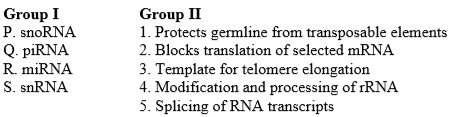Biotechnology - (BT) 2018 GATE Paper (Practice Test) - Question 39

Determine the correctness or otherwise of the following Assertion [a] and the Reason [r]

Assertion: Ab initio gene finding algorithms that predict protein coding genes in eukaryotic genomes are not completely accurate.

Reason: Eukaryotic splice sites are difficult to predict.

Biotechnology - (BT) 2018 GATE Paper (Practice Test) - Question 40

Which one of the following amino acids is catalyzed by activated macrophages to produce reactive nitrogen species?

Biotechnology - (BT) 2018 GATE Paper (Practice Test) - Question 41

Determine the correctness or otherwise of the following Assertion [a] and the Reason [r]

Assertion: The association constant in water for the G-C base pair is three times lower than that for the A-T base pair.

Reason: There are three hydrogen bonds in the G-C base pair and two in the A-T base pair.

Biotechnology - (BT) 2018 GATE Paper (Practice Test) - Question 42

Which one of the combinations of the following statements is true about antibody structure?

P. Limited proteolysis of rabbit IgG with the enzyme pepsin generates two antigen-binding regions (Fab) and an Fc fragment

Q. Limited proteolysis of rabbit IgG with the enzyme papain generates a single bivalent antigen-binding region F(ab')2  and peptide fragments

R. The Fc fragment of IgG can self-associate and crystallize into a lattice

S. The F(ab')2 fragment of IgG is composed of both light and heavy chains

Biotechnology - (BT) 2018 GATE Paper (Practice Test) - Question 43

Which one of the following statements is true with regard to processing and presentation of protein antigens?

Biotechnology - (BT) 2018 GATE Paper (Practice Test) - Question 44

Which of the following are true about bacterial superoxide dismutase?

P. Present in obligate aerobes
Q. Present in facultative anaerobes
R. Present in aerotolerant anaerobes
S. Absent in obligate aerobes

Biotechnology - (BT) 2018 GATE Paper (Practice Test) - Question 45

Which of the following are true with regard to anaerobic respiration in bacteria?

P.  The final electron acceptor is an inorganic substance other than molecular oxygen
Q.  The number of ATP molecules produced per glucose molecule is more than that produced in aerobic respiration
R.  The number of ATP molecules produced per glucose molecule is less than that produced in aerobic respiration
S.  Only substrate level phosphorylation is used to generate ATP

Biotechnology - (BT) 2018 GATE Paper (Practice Test) - Question 46

Shear stress versus shear rate behavior of four different types of fluids (I, II, III and IV) are shown in the figure below.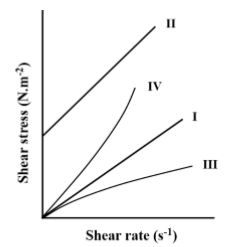Which one of the following options is correct?

Biotechnology - (BT) 2018 GATE Paper (Practice Test) - Question 47

An analysis of DNA-protein interactions was carried out using all DNA-protein complexes in the protein data bank (PDB). The frequency distribution of four amino acid residues, represented as P, Q, R and S, occurring in non-covalent interactions between the protein and DNA backbone is shown below.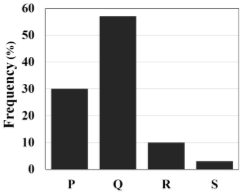Which one of the following is correct?

Biotechnology - (BT) 2018 GATE Paper (Practice Test) - Question 48

A pedigree of an inheritable disease is shown below.

What type of inheritance does the disease follow?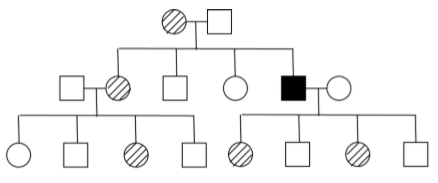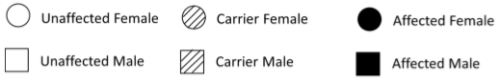Biotechnology - (BT) 2018 GATE Paper (Practice Test) - Question 49

Match the industrial products mentioned in Group I with their producer organisms in Group II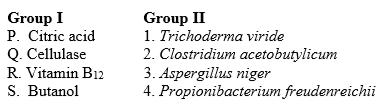Biotechnology - (BT) 2018 GATE Paper (Practice Test) - Question 50

5' capping of mRNA transcripts in eukaryotes involves the following events:

P. Addition of GMP on the 5' end

Q. Removal of g-phosphate of the triphosphate on first base at the 5' end

R. 5'-5' linkage between GMP and the first base at 5' end

S. Addition of methyl group to N7 position of guanine

Which one of the following is the correct sequence of events?

Biotechnology - (BT) 2018 GATE Paper (Practice Test) - Question 51

Calculate the following integral (up to two decimal places)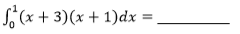Biotechnology - (BT) 2018 GATE Paper (Practice Test) - Question 52

The probability distribution for a discrete random variable �� is given below.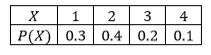The expectation value of �� is (up to one decimal place) _________

Biotechnology - (BT) 2018 GATE Paper (Practice Test) - Question 53

If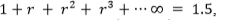then,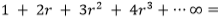(up to two decimal places) _________

Biotechnology - (BT) 2018 GATE Paper (Practice Test) - Question 54

Moist heat sterilization of spores at 121 oC follows first order kinetics as per the expression: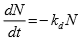where, N is the number of viable spores, t is the time, kd is the rate constant and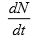is the rate of change of viable spores.
If kd value is 1.0 min-1, the time (in minutes) required to reduce the number of viable spores from an initial value of 1010 to a final value of 1 is (up to two decimal places) _________

Biotechnology - (BT) 2018 GATE Paper (Practice Test) - Question 55

An aqueous solution containing 6.8 mg/L of an antibiotic is extracted with amyl acetate. If the partition coefficient of the antibiotic is 170 and the ratio of water to solvent is 85, then the extraction factor is _________

Biotechnology - (BT) 2018 GATE Paper (Practice Test) - Question 56

A microbial strain is cultured in a 100 L stirred fermenter for secondary metabolite production. If the specific rate of oxygen uptake is 0.4 h-1 and the oxygen solubility in the broth is 8 mg/L, then the volumetric mass transfer coefficient (KLa) (in s-1) of oxygen required to achieve a maximum cell concentration of 12 g/L is (up to two decimal places) _________

Biotechnology - (BT) 2018 GATE Paper (Practice Test) - Question 57

In a chemostat, the feed flow rate and culture volume are 100 ml/h and 1.0 L, respectively. With glucose as substrate, the values of µmax and Ks are 0.2 h-1 and 1 g/L, respectively. For a glucose concentration of 10 g/L in the feed, the effluent substrate concentration (in g/L) is _________

Biotechnology - (BT) 2018 GATE Paper (Practice Test) - Question 58

Mammalian cells in active growth phase were seeded at a density of 1X105 cells/ml. After 72 hours, 1X106 cells/ml were obtained. The population doubling time of the cells in hours is (up to two decimal places) _________

Biotechnology - (BT) 2018 GATE Paper (Practice Test) - Question 59

Yeast converts glucose to ethanol and carbon dioxide by glycolysis as per the following reaction: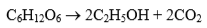Assuming complete conversion, the amount of ethanol produced (in g) from 200 g of glucose is (up to two decimal places) _________

Biotechnology - (BT) 2018 GATE Paper (Practice Test) - Question 60

At the end of a batch culture, glucose solution is added at a flow rate of 200 ml/h. If the culture volume after 2 h of glucose addition is 1000 ml, the initial culture volume (in ml) is _________

Biotechnology - (BT) 2018 GATE Paper (Practice Test) - Question 61

Consider the following alignment of two DNA sequences: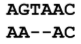Assuming an affine gap scoring scheme of an identity matrix for substitution, a gap initiation penalty of 1 and a gap extension penalty of 0.1, the score of the alignment is (up to one decimal place) _________

Biotechnology - (BT) 2018 GATE Paper (Practice Test) - Question 62

First order deactivation rate constants for soluble and immobilized amyloglucosidase enzyme are 0.03 min-1 and 0.005 min-1, respectively. The ratio of half-life of the immobilized enzyme to that of the soluble enzyme is (rounded off to the nearest integer) _________

Biotechnology - (BT) 2018 GATE Paper (Practice Test) - Question 63

Consider a simple uni-substrate enzyme that follows Michaelis-Menten kinetics. When the enzyme catalyzed reaction was carried out in the presence of 10 nM concentration of an inhibitor, there was no change in the maximal velocity. However, the slope of the Lineweaver-Burk plot increased 3-fold. The dissociation constant for the enzyme-inhibitor complex (in nM) is _________

Biotechnology - (BT) 2018 GATE Paper (Practice Test) - Question 64

The product of complete digestion of the plasmid shown below with EcoRI and HaeIII was purified and used as a template in a reaction containing Klenow fragment of DNA polymerase, dNTPs and [α-32P]-dATP in a suitable reaction buffer. The product thus obtained was purified and subjected to gel electrophoresis followed by autoradiography.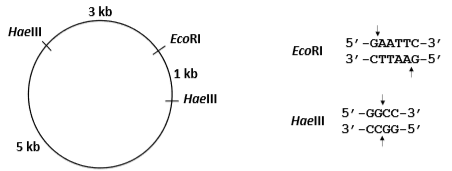The number of bands that will appear on the X-ray film is _________

Biotechnology - (BT) 2018 GATE Paper (Practice Test) - Question 65

A rod shaped bacterium has a length of 2 µm, diameter of 1 µm and density the same as that of water. If proteins constitute 15% of the cell mass and the average protein has a mass of 50 kDa, the number of proteins in the cell is _________ (1 Da = 1.6 X 10-24g)

## GATE Past Year Papers for Practice (All Branches)

407 docs|127 tests
 Use Code STAYHOME200 and get INR 200 additional OFF Use Coupon Code
Information about Biotechnology - (BT) 2018 GATE Paper (Practice Test) Page
In this test you can find the Exam questions for Biotechnology - (BT) 2018 GATE Paper (Practice Test) solved & explained in the simplest way possible. Besides giving Questions and answers for Biotechnology - (BT) 2018 GATE Paper (Practice Test), EduRev gives you an ample number of Online tests for practice

## GATE Past Year Papers for Practice (All Branches)

407 docs|127 tests